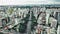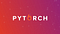# Rent price prediction using PyTorch — Part1: Data Cleaning

This is the first part of a series of tutorials for getting started with PyTorch. Along with these tutorials, we try to get familiar with some basic deep learning modelings.Photo by sergio souza on Unsplash

For the first part, we are going to get our data ready for applying models. We use datasets of rent prices for houses in Brazil.

So, let’s start. First read the data into a pandas dataframe:

Looking at the first five rows of dataframe, we can figure out that some of our features need to be converted to numerical values. …

# PyTorch tutorial for beginners — 5 functions that you probably didn’t know about

PyTorch is an open-source library developed by Facebook’s AI Research Lab. This Python-based scientific computing package is a replacement for NumPy library, which helps you with using the power of GPUs. PyTorch aims at giving researchers the flexibility and speed needed for their deep learning algorithms.Credits

In this tutorial, you will be familiar with some basic functions of PyTorch that you might not know before. Each section will have a look at a function of PyTorch related to Tensors. First, two examples will be explained and then a wrong code will be tested to break the function. So, we’ll be…## Ali Khatami

Software Engineer | PhD Student @ TU Delft | Website: akhatami.com